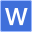2017年GRE考试数学考点讲解8

2017-2-21 15:56:00   无忧考网     [ 手机版 ] [下载Word文档 ]
GRE网权威发布《2017年GRE考试数学考点讲解8》（全文共659字），更多2017年GRE考试数学考点讲解8相关文档资源请访问无忧考网GRE留学外语考试频道。• 新东方金牌名师直冲高分！2017年GRE考试全程课程火热开售中>>
• 　　Coefficient

A constant multiplier of a variable is known as coefficient. They are typically represented by letters a,b,c.

Example:

In the expression 3x+5,3 is the coefficient of x.

In the equation 4x+2y=6,4 is the coefficient of x and 2 is the coefficient of y.

In the inequality 2*sin(x)+3>z,2 is the coefficient of the function sin(x).

Remember:

Coefficient cannot change its value.

GRE数学考点介绍：Multiplying Monomials

Multiplying Monomials

To multiply two monomials, multiply the coefficients and add the power of the variables.

By multiplying 3x and 4x, we get (4*3)x∧(1+1)=12x2.

By multiply 3x and 4y, we get (4*3)xy=12xy.

Remember:

Do not forget to add the powers of the same variable.

The terms need not be like terms to be multiplied.